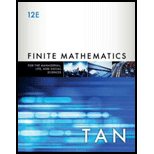Chapter 6.4, Problem 16E### Finite Mathematics for the Manager...

12th Edition
Soo T. Tan
ISBN: 9781337405782

#### Solutions

Chapter
Section### Finite Mathematics for the Manager...

12th Edition
Soo T. Tan
ISBN: 9781337405782
Textbook Problem

# In Exercises 1-22, evaluate the given expression. C ( 6 , 5 )

To determine

To evaluate:

The expression C(6,5).

Explanation

Approach:

The formula to calculate the number of combinations is given by,

C(n,r)=n!r!(nr)!

Here, n is the distinct object taken r at a time and rn.

Calculation:

C(6,5)=6!5!(

### Still sussing out bartleby?

Check out a sample textbook solution.

See a sample solution

#### The Solution to Your Study Problems

Bartleby provides explanations to thousands of textbook problems written by our experts, many with advanced degrees!

Get Started

## Additional Math Solutions

#### Find more solutions based on key concepts